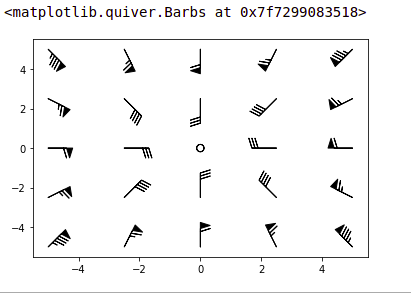Related Articles

# Matplotlib.pyplot.barbs() in Python

• Last Updated : 21 Apr, 2020

Matplotlib is a library of Python bindings which provides the user with a MATLAB-like plotting framework. Matplotlib can be used in Python scripts, the Python and IPython shell, web application servers, and various graphical user interface toolkits like Tkinter, awxPython, etc.

## Matplotlib.pyplot.barbs()

`matplotlib.pyplot.barbs` method is used to plot a 2D field of barbs.

### What are barbs?

Barbs are used majorly in meteorology to plot the speed and direction of winds, but can be used to plot any two-dimensional vector quantity. Barbs are able to provide more precise and quantitative information about vector magnitude when compared to arrows. This is mainly because arrows use their length to give a sense of the magnitude of the vector, while barbs use triangles or slanted lines as shown below:

```                   /\    \
/  \    \
/    \    \    \
/      \    \    \
------------------------------
```
• The largest increment in magnitude is denoted by a triangle (or flag)
• The smallest increment is a half-line

Syntax : matplotlib.pyplot.barbs(x_coordinate, y_coordinate, x_direction, y_direction, [colour])

Parameters:
x_coordinate : x-coordinate of the barb location
(may be a 2-dimensional array in case of multiple barbs)
y_coordinate : y-coordinate of the barb location
(may be a 2-dimensional array in case of multiple barbs)
x_direction : x-component of the direction of barb shaft
(may be a 2-dimensional array in case of multiple barbs)
y_direction : y-component of the direction of the barb shaft
(may be a 2-dimensional array in case of multiple barbs)
colour (optional): specifies the colour of the barb in the graph

Optional Parameters
length : length of the barb in points, other parts of the barb are scaled against this
pivot : part of the barb anchored to the grid; the barb rotates about this point. Maybe a number, which shifts the barb that many points away from the grid point

Return Value : Returns a 2D graph with arrows plotted

Note :

• If the x-coordinate and y-coordinate of the barb locations are not given, they will be generated as a uniform integer meshgrid based on x_direction and y_direction
• If x-coordinate and y-coordinate are one-dimensional but x_direction and y_direction are two-dimensional, then x-coordinate and y-coordinate would be expanded to a two-dimensional meshgrid using `x, y=numpy.meshgrid(x, y)`. In this case, the lengths of x-coordinate and y-coordinate must match the row and column dimensions of x_direction and y_direction

Sample Code:

 `import` `matplotlib.pyplot as plt``import` `numpy as np`` ` `x ``=` `np.linspace(``-``5``, ``5``, ``5``)``X, Y ``=` `np.meshgrid(x, x)``U, V ``=` `12` `*` `X, ``12` `*` `Y`` ` `data ``=` `[(``-``1.5``, .``5``, ``-``6``, ``-``6``),``        ``(``1``, ``-``1``, ``-``46``, ``46``),``        ``(``-``3``, ``-``1``, ``11``, ``-``11``),``        ``(``1``, ``1.5``, ``80``, ``80``),``        ``(``0.5``, ``0.25``, ``25``, ``15``),``        ``(``-``1.5``, ``-``0.5``, ``-``5``, ``40``)]`` ` `data ``=` `np.array(data, dtype``=``[(``'x'``, np.float32),``                             ``(``'y'``, np.float32),``                             ``(``'u'``, np.float32), ``                             ``(``'v'``, np.float32)])`` ` `plt.barbs(X, Y, U, V)`

Output :Attention geek! Strengthen your foundations with the Python Programming Foundation Course and learn the basics.

To begin with, your interview preparations Enhance your Data Structures concepts with the Python DS Course. And to begin with your Machine Learning Journey, join the Machine Learning – Basic Level Course

My Personal Notes arrow_drop_up